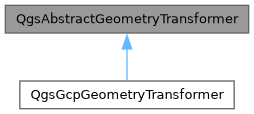QGIS API Documentation  3.23.0-Master (7c4a6de034)
QgsAbstractGeometryTransformer Class Referenceabstract

An abstract base class for classes which transform geometries by transforming input points to output points. More...

#include <qgsgeometrytransformer.h>

Inheritance diagram for QgsAbstractGeometryTransformer:[legend]

Public Member Functions

virtual ~QgsAbstractGeometryTransformer ()=default

virtual bool transformPoint (double &x, double &y, double &z, double &m)=0
Transforms the point defined by the coordinates (x, y, z) and the specified m value. More...

Detailed Description

An abstract base class for classes which transform geometries by transforming input points to output points.

Since
QGIS 3.18

Definition at line 32 of file qgsgeometrytransformer.h.

◆ ~QgsAbstractGeometryTransformer()

 virtual QgsAbstractGeometryTransformer::~QgsAbstractGeometryTransformer ( )
virtualdefault

◆ transformPoint()

 virtual bool QgsAbstractGeometryTransformer::transformPoint ( double & x, double & y, double & z, double & m )
pure virtual

Transforms the point defined by the coordinates (x, y, z) and the specified m value.

Parameters
 x point x coordinate y point y coordinate z point z coordinate, or NaN if the input point is 2D only m point m value, or NaN if not available
Returns
true if point was transformed (or no transformation was required), or false if point could not be transformed successfully.

Example

A transformer which multiples the x coordinate by 3 and adds 10 to the y coordinate:

class MyTransformer(QgsAbstractGeometryTransformer):
def transformPoint(self, x, y, z, m):
# returns a tuple of True to indicate success, then the modified x/y/z/m values
return True, x*3, y+10, z, m
An abstract base class for classes which transform geometries by transforming input points to output ...

Implemented in QgsGcpGeometryTransformer.

The documentation for this class was generated from the following file: